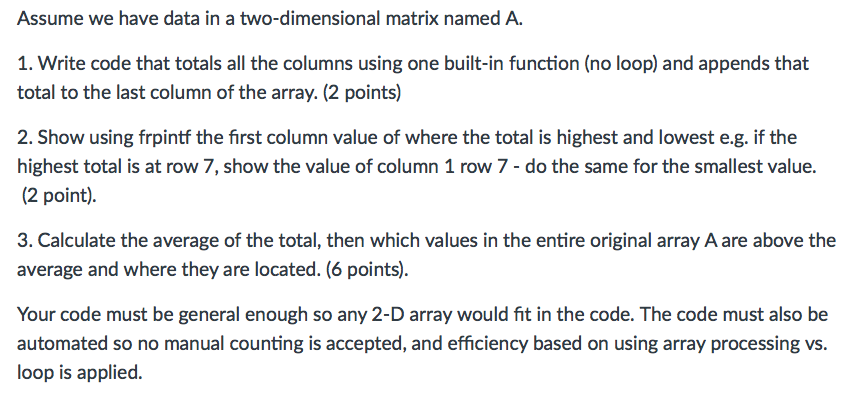# Answered! Assume we have data in a two – dimensional matrix named A. Write code that totals all the columns using one built-…Assume we have data in a two – dimensional matrix named A. Write code that totals all the columns using one built-in function (no loop) and appends that total to the last column of the array. Show using frpintf the first column value of where the total is highest and lowest e.g. if the highest total is at row 7, show the value of column 1 row 7 – do the same for the smallest value. Calculate the average of the total, then which values in the entire original array A are above the average and where they are located. Your code must be general enough so any 2 – D array would fit in the code. The code must also be automated so no manual counting is accepted, and efficiency based on using array processing vs. loop is applied.

1.

A = [1 3;4 5]
B=sum(A)
B=B’
A = [A B]

Don't use plagiarized sources. Get Your Custom Essay on
Answered! Assume we have data in a two – dimensional matrix named A. Write code that totals all the columns using one built-…
GET AN ESSAY WRITTEN FOR YOU FROM AS LOW AS \$13/PAGE

sum(A) sums the column of 2 dimentional matrix and give answer in 1×2 matrix i.e. row

B=B’ converts the result row to column

A = [A B] appends the column to the given two dimetional matrix

But I think the question should be “Write code that totals all the rows…” instead of “Write code that totals all the columns…” Please confirm as the other two answers are based on that.

Assuming it is row, the answer would be as follow

1.

A = [1 3;4 5;4 7; 3 0; 2 8; 9 3]
% get the total of each row
total = sum(A,2)
%append the total column in the original array
B = [A total]

2

% Find the highest value in the total array
[M,I] = max(total)
% get the column 1 for the highest total and display result on screen
fprintf(‘Coulmn 1 value for highest total row %d’, I)
fprintf (‘ is %dn’, A(I,1))

% Find the smallest value in the total array
[M,I] = min(total)
% get the column 1 for the smallest total and display result on screen
fprintf(‘Coulmn 1 value for smallest total row %d’, I)
fprintf (‘ is %dn’, A(I,1))

3

% get the average of the total
avg = mean(total)
%find the location of the value above average
C=find(A > avg)
%find all the values above average
A(C)